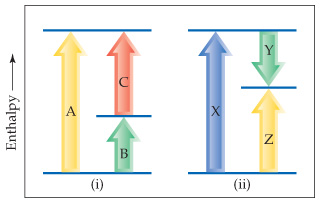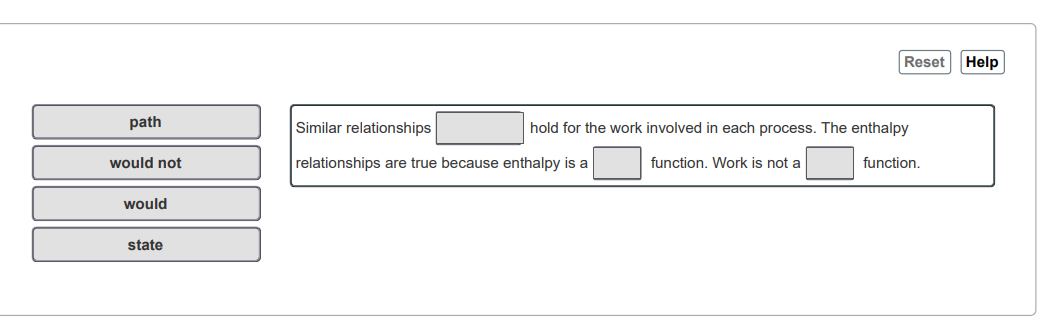# Problem: Consider the two diagrams below.Would similar relationships hold for the work involved in each process? Why?  Match the words in the left column to the appropriate blanks in the sentences on the right

###### FREE Expert Solution

Recall that:

$\mathbf{∆}{\mathbf{H}}_{\mathbf{rxn}}\mathbf{=}\mathbf{∆}{\mathbf{H}}_{\mathbf{f}\mathbf{,}\mathbf{products}}\mathbf{-}\mathbf{∆}{\mathbf{H}}_{\mathbf{f}\mathbf{,}\mathbf{reactants}}$

• Enthalpy → can be computed based on initial and final states
• State function → property of a system that does not depend on the path taken
• Work →  depends on the path taken
87% (470 ratings)###### Problem Details

Consider the two diagrams below.Would similar relationships hold for the work involved in each process? Why?  Match the words in the left column to the appropriate blanks in the sentences on the rightFrequently Asked Questions

What scientific concept do you need to know in order to solve this problem?

Our tutors have indicated that to solve this problem you will need to apply the First Law of Thermodynamics concept. You can view video lessons to learn First Law of Thermodynamics. Or if you need more First Law of Thermodynamics practice, you can also practice First Law of Thermodynamics practice problems.